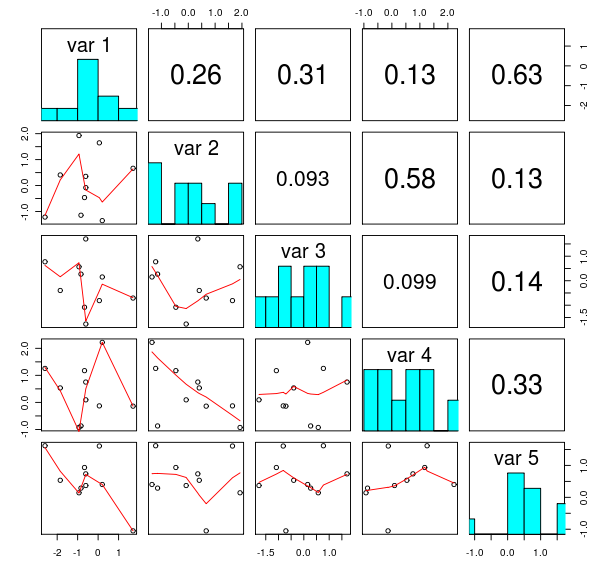# matplotlib analog of R's `pairs`

### Question

R has a useful function `pairs` that provides nice matrix of plots of pairwise connections between variables in a data set. The resulting plot looks similar to the following figure, copied from this blog post:Is there any ready to use function based on python's matplolib? I have searched its gallery, but couldn't find anything that resembles what I need. Technically, this should be a simple task, but proper handling of all the possible cases, labels, titles, etc is very tedious.

UPDATE see below my answer with a quick and dirty approximation.

1
34
2/8/2017 2:24:13 PM

`Pandas` has a built in function `scatter_matrix` (source code) which is something like this.

``````import numpy as np
import pandas as pd
import matplotlib.pyplot as plt

df = pd.DataFrame(np.random.randn(1000, 4), columns=['A','B','C','D'])
axes = pd.tools.plotting.scatter_matrix(df, alpha=0.2)
plt.tight_layout()
plt.savefig('scatter_matrix.png')
``````However it is `pandas` specific (but could be used as a starting point).

There are some more `R` like plots in pandas. Have a look at the docs.

46
10/18/2012 2:25:29 PM

Quick and dirty approximation to my needs:

``````def pair(data, labels=None):
""" Generate something similar to R `pair` """

nVariables = data.shape
if labels is None:
labels = ['var%d'%i for i in range(nVariables)]
fig = pl.figure()
for i in range(nVariables):
for j in range(nVariables):
nSub = i * nVariables + j + 1
if i == j:
ax.hist(data[:,i])
ax.set_title(labels[i])
else:
ax.plot(data[:,i], data[:,j], '.k')

return fig
``````

The code above is hereby released into the public domain